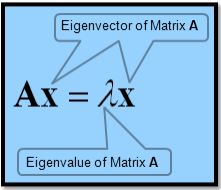# How to find the Eigenvalues and Eigenvectors of a square matrix using SciPy?

We can find the Eigenvalues and Eigenvectors for a square matrix, say A, with the help of following relation −SciPy library has scipy.linalg.eig() function for computing the eigenvalues and eigenvectors of a square matrix.

Let’s understand how we can use this function to calculate the inverse of a matrix −

## Example

Eigenvalues and Eigenvectors of a 2 by 2 matrix

#Importing the scipy package
import scipy

#Importing the numpy package
import numpy as np

#Declaring the numpy array(Square Matrix)
A = np.array([[3,3.5],[3.2,3.6]])

#Passing the values to scipy.linalg.eig() function
eigvalues, eigvectors = scipy.linalg.eig(A)
#Printing the result for eigenvalues
print(eigvalues)
#Printing the result for eigenvectors
print(eigvectors)

## Output

[-0.06005952+0.j 6.66005952+0.j]
[[-0.75283678 -0.6911271 ]
[ 0.65820725 -0.72273324]]

## Example

Eigenvalues and Eigenvectors of a 3 by 3 matrix

import scipy
import numpy as np
A = np.array([[2,1,-2],[1,0,0],[0,1,0]])
eigvalues, eigvectors = scipy.linalg.eig(A)
print(eigvalues)
print(eigvectors)

## Output

[-1.+0.j 2.+0.j 1.+0.j]
[[-0.57735027 -0.87287156 0.57735027]
[ 0.57735027 -0.43643578 0.57735027]
[-0.57735027 -0.21821789 0.57735027]]